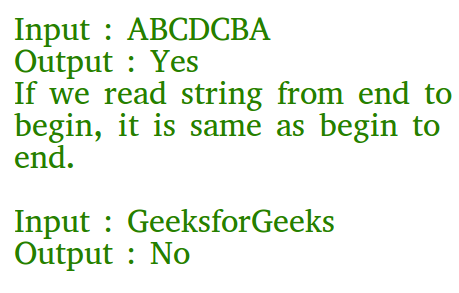Open in App
Not now

# C Program to Check if a Given String is Palindrome

• Difficulty Level : Easy
• Last Updated : 16 Feb, 2023

A string is said to be palindrome if the reverse of the string is the same as the string. For example, “abba” is a palindrome because the reverse of “abba” will be equal to “abba” so both of these strings are equal and are said to be a palindrome, but “abbc” is not a palindrome.

There are multiple methods to check palindromic strings such as:

• Using the standard (simple) method
• Using function in C
• Using String library function of reverse and compare in C
• Using RecursionRecommended Practice

## 1. Using the Standard (simple) Method

Algorithm:

• Initialize 2 variables, l from the start and h from the end.
• now while (h>l), we will check its equivalent character in the string.
• if it’s not equal then it’s not a palindrome
• else it will traverse half character and the string is a palindrome.

## C

 `// C implementation to check if a given ``// string is palindrome or not``#include ``#include `` ` `int` `main()``{``    ``char` `str[] = { ``"abbba"` `};`` ` `    ``// Start from leftmost and ``    ``// rightmost corners of str``    ``int` `l = 0;``    ``int` `h = ``strlen``(str) - 1;`` ` `    ``// Keep comparing characters``    ``// while they are same``    ``while` `(h > l) {``        ``if` `(str[l++] != str[h--]) {``            ``printf``(``"%s is not a palindrome\n"``, str);``            ``return` `0;``            ``// will return from here``        ``}``    ``}`` ` `    ``printf``(``"%s is a palindrome\n"``, str);`` ` `    ``return` `0;``}`

Output

```abbba is a palindrome
```

Time complexity: O(n)
Auxiliary Space: O(1)

## 2. Using function in C

Algorithm of isPalindrome(str) function:

1. Find the length of str. Let length be n.
2. Initialize low and high indexes as 0 and n-1 respectively.
3. Do following while low index ‘l’ is smaller than high index ‘h’.
•  If str[l] is not same as str[h], then return false.
• Increment l and decrement h, i.e., do l++ and h–.
4. If we reach here, it means we didn’t find a mis

## C

 `// C program to check if a string is ``// a palindrome or not.``#include ``#include `` ` `// A function to check if a``// string str is palindrome``void` `isPalindrome(``char` `str[])``{``    ``// Start from leftmost and ``    ``// rightmost corners of str``    ``int` `l = 0;``    ``int` `h = ``strlen``(str) - 1;`` ` `    ``// Keep comparing characters ``    ``// while they are same``    ``while` `(h > l)``    ``{``        ``if` `(str[l++] != str[h--])``        ``{``            ``printf``(``"%s is not a palindrome\n"``, str);``            ``return``;``        ``}``    ``}``    ``printf``(``"%s is a palindrome\n"``, str);``}`` ` `// Driver program to test above function``int` `main()``{``    ``isPalindrome(``"abba"``);``    ``isPalindrome(``"abbccbba"``);``    ``isPalindrome(``"geeks"``);``    ``return` `0;``}`

Output

```abba is a palindrome
abbccbba is a palindrome
geeks is not a palindrome
```

Time complexity: O(n)
Auxiliary Space: O(1)

## 3. Using String Library Function Compare in C

We can also check a palindromic string with the help of compare function in the C library. Given string is reversed and then it is compared with the help of strcmp() which compares two strings in C. If they are equal then the string will be a palindromic string else not.

## C

 `// C program to check palindromic string by reversing and``// comparing``#include ``#include `` ` `int` `main()``{``    ``char` `str;``    ``printf``(``"Enter String (upto 20 characters length): "``);``    ``scanf``(``"%s"``, str);`` ` `    ``int` `strSize = ``strlen``(str);``    ``if` `(strSize > 20) {``        ``printf``(``"\nError: strSize should be less than 20 "``               ``"characters."``);``        ``return` `0;``    ``}`` ` `    ``// reverse 'str' and store in 'strReversed'``    ``char` `strReversed;``    ``for` `(``int` `i = strSize - 1; i >= 0; --i) {``        ``strReversed[strSize - 1 - i] = str[i];``    ``}`` ` `    ``// comparing string, if they are equal then it's a``    ``// palindromic string.``    ``if` `(``strcmp``(str, strReversed) == 0) {``        ``printf``(``"\n%s is a palindrome"``, str);``    ``}``    ``else` `{``        ``printf``(``"\n%s is not a palindrome"``, str);``    ``}``    ``return` `0;``}`

Output:

```Enter String : abba
abba is a palindrome```

Time complexity: O(n)
Auxiliary Space: O(1)

## 4. Using Recursion

With the help of recursion, we can check the palindromic string in C. In ispalindrome() function lower variable i.e, l is increased and the higher variable i.e, r is decreased and if at any point of time they are not equal then it means the string is not a palindromic string else it will continue till (l>=r) and if all the indices of l and r are equal then we can say that the string is a palindromic string and we will return 1.

## C

 `// C program to check palindromic string by recursion``#include ``#include `` ` `int` `isPalindrome(``char` `*str, ``int` `l, ``int` `r){``     ``if``(NULL == str || l < 0 || r < 0){``         ``return` `0;``     ``}``     ``if``(l >= r)``         ``{``             ``return` `1;``         ``}``     ``if``(str[l] == str[r]){``         ``return` `isPalindrome(str, l + 1, r - 1);``     ``}``     ``return` `0;`` ``}`` ` `int` `main()``{``    ``char` `s[] = {``"abbba"``} ;  ``    ``int` `in=0;``    ``int` `i=0,n=``sizeof``(s)/``sizeof``(s);``     ` `    ``if``(isPalindrome(s,i,``strlen``(s) - 1))``         ``printf``(``"%s is palindrome"``,s);``    ``else``        ``printf``(``"%s is not palindrome"``,s);`` ` ` ``}`

Output

`abbba is palindrome`

Time complexity: O(n)
Auxiliary Space: O(n), For recursion stack

My Personal Notes arrow_drop_up